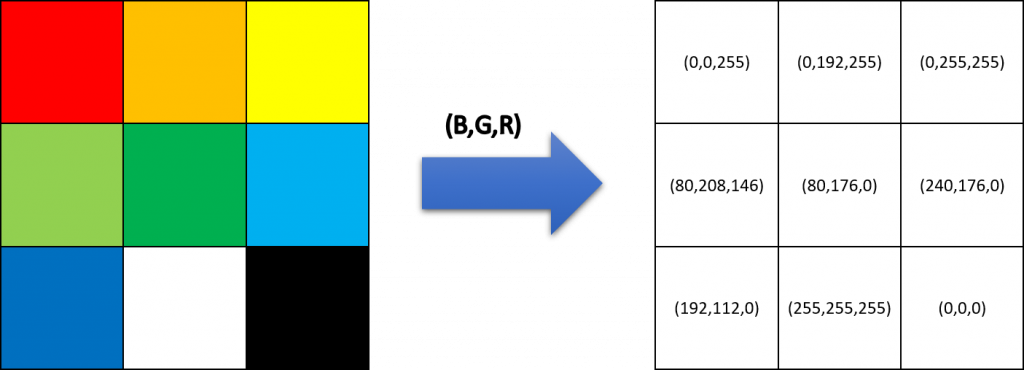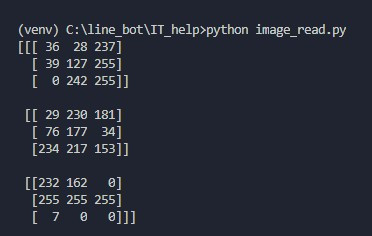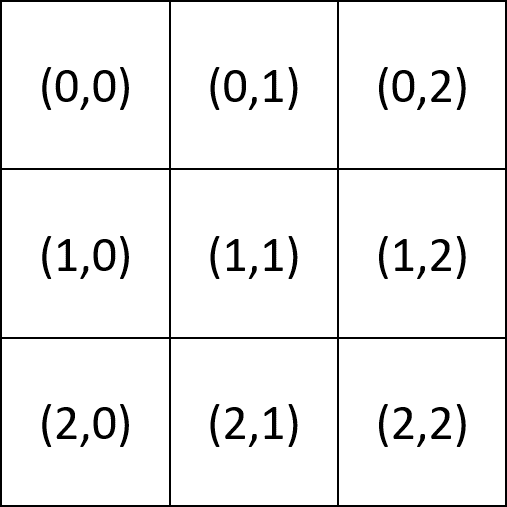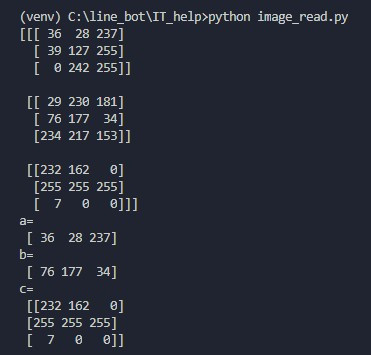DAY 11
0

安裝OPENCV

pip install opencv-python opencv-contrib-python
pip install numpy

關於圖片的顏色

R=紅色，G=綠色，B=藍色，是光的三原色，pic=[[[0,0,255],[0,192,255],[0,255,255]],
[[80,208,146],[80,176,0],[240,176,0]],
[[192,112,0],[255,255,255],[0,0,0]]]import cv2 as cv
import numpy as np

print(img)

print出來的結果會像是這樣：關於圖片的位置import cv2 as cv
import numpy as np

print(img)

a = img[0,0]
b = img[1,1]
c = img[2,0:3]

print('a=\n',a)
print('b=\n',b)
print('c=\n',c)0